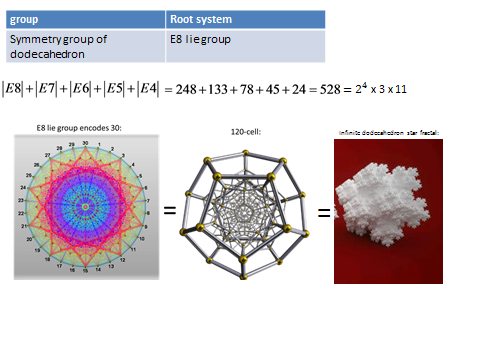top of page
Search

# Root system of dodecahedron E8 lie groupthe root system of the 120-cell is the infinite dimensional E8 lie group fractal which is the infinite tetrahedron grid which is energy meaning it is a kugalblits and the superstring E8xE8(xen particle) and its frequency is 528hzThis shows the E8 lie group is energy(kugalblits) and the energy is the astral plane which is the infinite tetrahedron grid (xen particle)